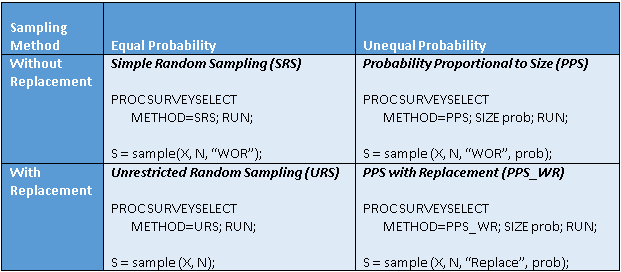Many simulation and resampling tasks use one of four sampling methods. When you draw a random sample from a population, you can sample with or without replacement. At the same time, all individuals in the population might have equal probability of being selected, or some individuals might be more likely than others. Consequently, the four common sampling methods are shown in the following 2 x 2 table.In SAS, the SURVEYSELECT procedure is a standard way to generate random samples. The previous table names of the four sampling methods, summarizes how to generate samples by using the SURVEYSELECT procedure in SAS/STAT, and shows how to use the SAMPLE function in SAS/IML.

The documentation for the SURVEYSELECT procedure uses terms that might not be familiar to programmers who are not survey statisticians. To help eliminate any confusion, the following sections describe the four common sampling methods and the corresponding METHOD= option in PROC SURVEYSELECT.

### Sampling without replacement

When you sample without replacement, the probability of choosing each item changes after each draw. The size of the sample cannot exceed the number of items.

Simple random sampling (SRS) means sampling without replacement and with equal probability. Dealing cards from a 52-card deck is an example of SRS. Use the METHOD=SRS option in PROC SURVEYSELECT to request simple random sampling.

Probability proportional to size (PPS) means sampling without replacement and with unequal probability. The classic example is counting the colors in a sample of colored marbles drawn from an urn that contains colors in different proportions. Use the METHOD=PPS option in PROC SURVEYSELECT to request PPS sampling and specify the relative sizes (or the probability vector) of items by using the SIZE statement.

### Sampling with replacement

When you sample with replacement, the probability of choosing each item does not change. The size of the sample can be arbitrarily large.

Unrestricted random sampling (URS) means sampling with replacement and with equal probability. Rolling a six-sided die and recording the face that appears is an example of URS. Use the METHOD=URS option in PROC SURVEYSELECT to request unrestricted random sampling.

Probability proportional to size with replacement means sampling with replacement and with unequal probability. An example is tossing two dice and recording the sum of the faces. Use the METHOD=PPS_WR option in PROC SURVEYSELECT to request PPS sampling with replacement. Use the the SIZE statement to specify the relative sizes or the probability vector for each item.

These four sampling methods are useful to the statistical programmer because they are often used in simulation studies. For more information about using the SAS DATA step and PROC SURVEYSELECT for basic sampling, see "Selecting Unrestricted and Simple Random with Replacement Samples Using Base SAS and PROC SURVEYSELECT (Chapman 2012)." PROC SURVEYSELECT contains many other useful sampling methods. For an overview of more advanced methods, see "PROC SURVEYSELECT as a Tool for Drawing Random Samples" (Lewis 2013).

The post Four essential sampling methods in SAS appeared first on The DO Loop.